## Starting Methods of Induction Motor:

Starting Methods of Induction Motor – At the time of starting the motor slip being unity, the load resistance with reference to the approximate circuit model of Fig. 9.23 is meaning thereby that short-circuit conditions prevail.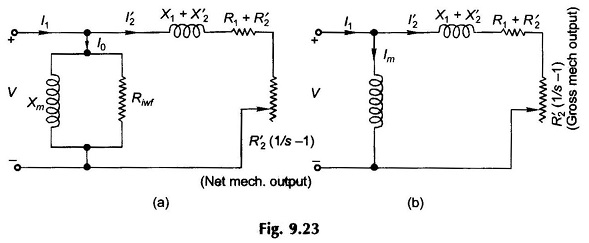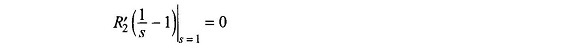Therefore, the motor current at starting can be as large as five to six times the full-load current. In comparison the exciting current in the shunt branch of the circuit model can be neglected reducing the circuit to that of Fig. 9.31.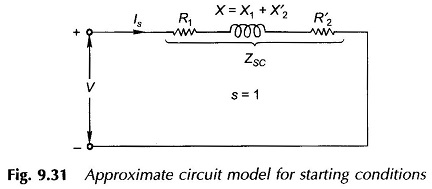Now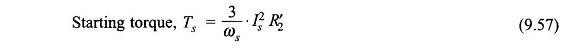Assuming for simplicity that as a rough approximation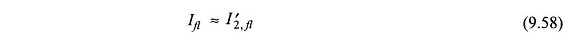i.e., the magnetizing current is neglected even under full-load conditions.

Then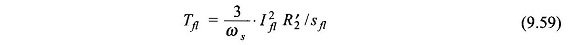where

The starting torque expressed as ratio of the full-load torque is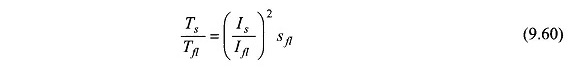### Starting Methods of Squirrel Cage Induction Motors:

Direct starting : When a squirrel-cage motor is started ‘direct-on-line,’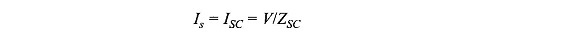i.e. starting current equals the short-circuit (blocked-rotor) current. LetThen from Eq. (9.60)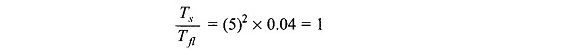This means that when the starting current is as large as five times the full-load current, the Starting torque just equals the full-load torque. With such a large starting current, the motor must accelerate and reach normal speed quickly otherwise overheating may damage the motor. The load on the motor at the time of starting must, therefore, be very light or preferably the motor must be on no-load.

To protect their supply systems, the electrical utilities have regulations against short-time current peaks; the consumer is heavily penalized through suitable tarrif for such peaks. Therefore, it is only small-size motors that can be started direct on­line. A bulk consumer can start motors up to 10 kW direct-on-line as long as he arranges to stagger the starting of such motors.

Reduced voltage starting : The starting current can be reduced to a tolerable level by reduced-voltage starting. This causes the starting torque to reduce heavily as it is proportional to the square of voltage. Such starting can only be carried out on no or light-load. Various methods of reduced voltage starting are discussed below.

Stator-impedance starting : Inclusion of resistors or inductors in the three lines feeding the stator of the Induction Motor reduces the stator terminal voltage to x V of the rated voltage V. The initial starting current is then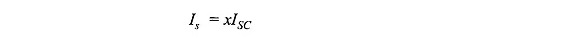Substituting in Eq. (9.60)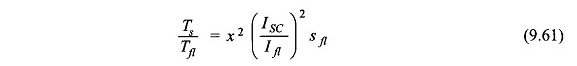Thus, while the starting current reduces by a fraction x of the rated-voltage starting current (ISC), the starting torque is reduced by a fraction x2 of that obtainable with direct switching. This method can be used for small motors such as those driving centrifugal pumps; but star-delta starting (given later) is cheaper with better starting torque.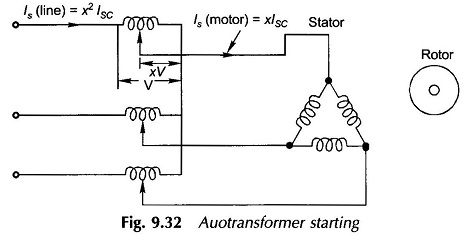Autotransformer starting : Reduced voltage for starting can be obtained from three autotransformers connected in star as shown in the schematic diagram of Fig. 9.32. If the voltage is reduced to a fraction x of the rated voltage V, the motor starting current (initial) is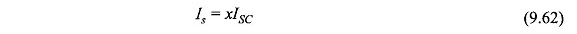where

• ISC = starting current (line) with full-voltage

The current drawn from the supply is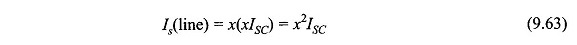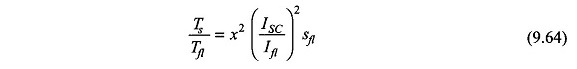It is found that while the starting torque is reduced to a fraction x2 of that obtainable by direct starting, the starting line current is also reduced by the same fraction. Compared to stator-impedance starting, the line current reduces further by a fraction x while torque remains the same. The autotransformer starting is much superior to the stator-impedance starting. Further, smooth starting and high acceleration are possible by gradually raising the voltage to the full line value.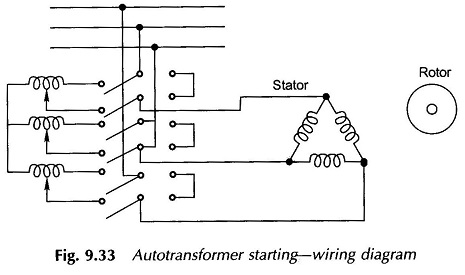After starting, the autotransformer is cut as shown in the wiring diagram of Fig. 9.33. It is to be observed that the autotransformer can be short-time rated. The use of an autotransformer is an expensive way of the Starting Methods of Induction Motor and is warranted for large motors only.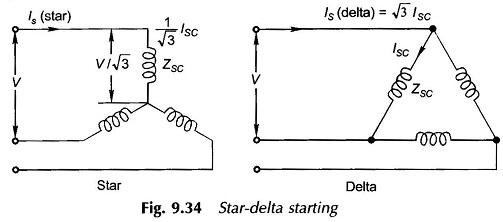Star-delta starting : The star-delta starting is an inexpensive two-step Starting Methods of Induction Motor. The motor designed for delta running is started across full-line voltage by connecting the phases in star as shown in the schematic diagram of Fig. 9.34.

In direct delta starting: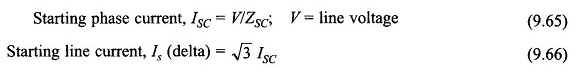In star starting:

Starting line (phase) current, Is (star)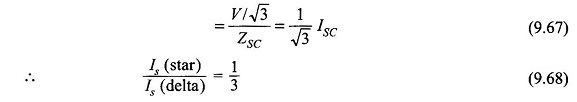Using Eq. (9.60)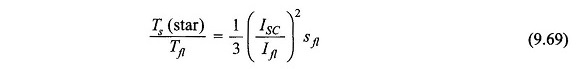where

• ISC = starting phase current (delta) and
• Ifl = full-load phase current (delta).

It is thus seen that star-delta starting reduces the starting torque to one-third that obtainable by direct-delta starting and also the starting line current to one-third. It just acts like autotransformer starting with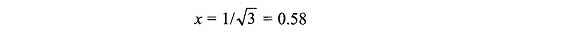A star-delta starter is much cheaper than an autotransformer starter and is commonly employed for both small and medium-size motors. The wiring diagram of star-delta starting is shown in Fig. 9.35.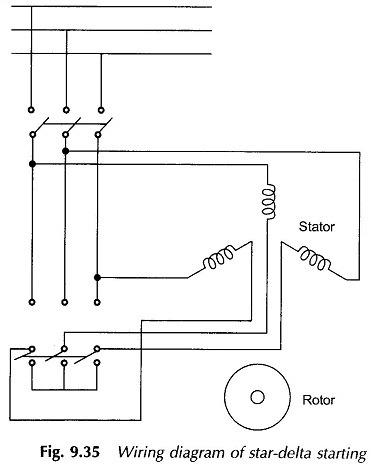### Starting of Slip Ring Induction Motor (Rotor Resistance Starting):

It has already been discussed that in slip-ring motors the starting current is reduced and the starting torque simultaneously increased by adding an external resistance in the rotor circuit.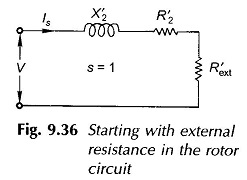Figure 9.36 shows the motor circuit (magnetizing branch neglected) with external resistance added in the rotor circuit. For simplicity, the stator impedance is also neglected. Here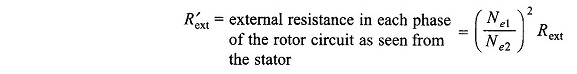where

• Rext = actual external resistance in the rotor circuit

Now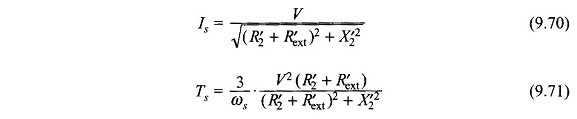Maximum starting torque is achieved at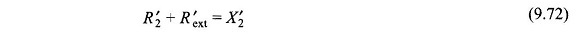which equals the breakdown torque orBy a suitable choice of Rext, the starting current and torque can be both adjusted to desirable levels. Since the maximum starting torque achievable is much more than the full-load torque, the use of slip-ring motors with rotor-resistance starting is ideal for starting on load. The external rotor resistance is arranged in steps which are gradually cut out during starting.

Scroll to Top